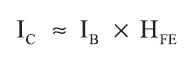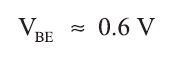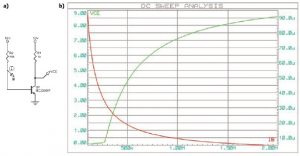Archive Article

# Bipolar Transistor BiasingGoing back to the basics is never a bad idea. Many electronics engineers are fluent with complex systems—such as microcontrollers, embedded OSes, or FPGAs—but seem to have more difficulties with single transistors. What a shame! A transistor can be a more adequate and cost-effective solution than an IC in many projects. Moreover, understanding what’s going on with simple parts can’t hurt, and transistors can even be fun! That’s why this month I will provide a refresher on how to use a one-cent bipolar junction transistor (BJT) to build an amplifier.

## OUR FRIEND, THE TRANSISTOR

The BJT is an old invention. In 1947, it was discovered at the Bell Laboratories by Walter H. Brattain and John Bardeen, who were on William Shockley’s team. The BJT comes in two flavors, NPN and PNP. For simplicity, I will focus on the NPN version. However, by reversing the power supply rails, everything would be applicable to its PNP cousin. BJT transistors have three terminals: collector (C), emitter (E), and base (B). Due to their internal semiconductor structure, the currents circulating through each of these terminals, as well as the voltages between them, are all linked together.

Let’s focus on the basic “common emitter” circuit (see Figure 1). With this setting, the emitter is grounded. There are two basic rules.

First, the current circulating through the collector is roughly proportional to the current applied on the base. Their ratio is the transistor current gain, which is indicated in the transistor’s datasheet and often noted ßF or HFE:Second, the voltage between the base and the emitter is stable and close to 0.6 V for more devices, as with any bipolar diode:Here’s how it works: If the voltage applied between the base and the emitter is lower than this threshold, then the transistor is blocked and no current circulates through the collector. If this voltage is increased to the threshold, then the transistor becomes active. You will not be able to increase the base voltage significantly above 0.6 V and the device will start to be current-controlled. In this mode, a given current will circulate through the base. The current through the collector will always be HFE times higher.

For example, if you have a transistor with a gain of 100 and inject 1 mA in the base, then 100 mA will flow through the collector. Of course, this is an approximate explanation, as the transistor’s physics are a little more complex, but is enough for my example. (Search for “Ebers-Moll model” online if you are interested in the details. Wikipedia also provides a good BJT summary.)

The examples in this article are based on the old faithful Fairchild Semiconductor BC238B transistor, but you could use any common NPN transistor (e.g., the ubiquitous 2N2222, the 2N3904, or the BC847 if you prefer surface-mount packages). Figure 2 shows a reproduction of the BC238B’s key characteristics from its datasheet. Figure 2a shows you the relationship between the voltage between the collector and the emitter (VCE) and the current through the collector (IC). Each curve corresponds to a given base current (IB). Look for an example at the curve for IB = 200 µA. As soon as the VCE voltage is above a couple of volts, the current circulating through the collector is nearly constant, around 50 mA. This means this specific transistor’s current gain is 50 mA divided by 200 µA, which is 250. Figure 2b shows the base voltage VBE. It is not strictly constant, but still close to 0.6 V, as explained.

A final but important point about BJT characteristics: Their current gain is far from precise. First, there is a huge dispersion on the current gain from transistor to transistor, even from the same manufacturing batch. Second, this gain will change with the transistor operating conditions and with the junction temperature in particular. Table 1 shows the specified gain for the BC238 family. It can range from 180 to 460 for the BC238B variant! The designer must take this difficulty into consideration.

## BASIC BIASING

Simulating a transistor is straightforward using a linear circuit simulator (e.g., SPICE), even if you prefer to wire it. I used Labcenter Electronics’s Proteus VSM in my example, but you can use any SPICE tool (e.g., Linear Technology’s free LTSpice) or an online version (e.g., CircuitLab, PartSim, etc.).

Figure 3 shows a basic circuit built around a BC238B. I connected the collector to a 10-VDC power source through a 1-kΩ resistor and used a 1-MΩ resistor between the transistor’s base and the 10-V power supply. The voltage applied on the base is above the 0.6-V threshold so the transistor will conduct. As discussed, the base voltage will stay close to the 0.6-V threshold (in fact, its simulated value is 0.66 V). The current circulating through the base could then be easily calculated by Ohm’s law applied on the base resistor: I = U/R = (10 – 0.66 V)/1 MΩ = 9.34 µA. You can then calculate the current circulating through the collector by multiplying this value by the transistor’s current gain, or the simulator can calculate it for you.

Take another look at Figure 3. The calculated collector current is 2.9 mA, which is 310 times higher than the base current. The BC238B model used by my SPICE variant seems to have a 310 gain. Consequently, the voltage drop across the collector resistor is U = R × I = 1 kΩ × 2.9 mA = 2.9 V. As the power supply voltage is 10 V, the voltage between the transistor’s collector and the ground should be 7.1 V (i.e., 10 – 2.9 V), as simulated.

Now imagine you want to use this BC238B transistor to build an AC signal amplifier (e.g., a small audio amplifier). Start with the schematic shown in Figure 3 and add the input AC signal on the transistor’s base. This input signal will periodically increase or decrease the current already applied on the base by the 1-MΩ resistor. These fluctuations will be amplified by the transistor’s current gain. Consequently, the collector voltage will fluctuate more than the input and you will have a working amplifier.

How can you design it? The first step is to define the so-called “transistor quiescent point” (i.e., you should first define the transistor’s behavior without an applied input signal). You will usually start by defining the collector resistor’s value based on the desired output impedance. Then you will need to calculate the resistor between the base and the power supply rail to set the transistor output to your desired DC value.

The rule is simple. For minimum distortion and clipping, you need to set the DC output voltage to half the supply voltage. In Figure 3, I used a 1-MΩ base resistor and found 7.1 V on the output (10 V/2 = 5 V would be preferable). Reducing the base resistor’s value will increase the base current, which will then reduce the mean output voltage (see Figure 4). This simulation shows that a base resistor close to 560 kΩ provides an average voltage on the output of 5 V, which is what we were looking for. The standby current through the collector is I = U/R = 5 V/1 kΩ = 5 mA.Figure 4
This simulation shows the collector-to-emitter voltage (green) and base current (red) when the base resistor value changes. There is an intermediate value, close to 560 kΩ, where the collector-to-emitter voltage is close to VCC/2, which is 5 V.

Now you have a correctly DC-biased transistor and you just have to inject the signal input on the base through a decoupling capacitor and extract the collector’s output signal through another decoupling capacitor (see Figure 5). The value of these capacitors are directly linked to the lowest frequency you want to amplify. You can either calculate it (remembering that a capacitor’s impedance is Z = 1/2πfC) or simulate it.Figure 5
A fixed-bias amplifier is simply build by injecting the input AC signal on the base through a capacitor. The time-domain simulation (top right) shows that the output voltage is close to ±1.6 VPP with ±10 mV on the input. The pass band extents down to 100 Hz (bottom left), while the distortion stays close to 1% with a second harmonic 25 dB lower than the signal (bottom right).

A 1-µF capacitor provides a reasonable 100-Hz low-frequency cutoff, as shown on the frequency response simulation. I also performed a time-domain simulation with a 20-mVPP, 1.2-kHz input signal. As shown in Figure 5, the resulting simulated output voltage is 3.2 VPP, providing a gain of 160. So you do have an amplifier.
Note that its voltage gain is not identical to the transistor’s current gain (remember, we got 160 against 300). This voltage gain is always lower relative to the HFE current gain, basically because you are adding a voltage and not a current on the base. The relationship between the two is not straightforward. Search for “hybrid-pi model” online if you need more explanation, or just simulate it.

## EMITTER-STABILIZED BIASING

You have now used just a basic transistor, two resistors, and two capacitors to design an AC amplifier with a fairly high gain. This is the so-called fixed-bias solution. But can you guess the problem with this? Remember that the transistor’s current gain is never well defined, except if you measure it yourself for each transistor and take care of the transistor’s operating condition and temperature.
Imagine you build the circuit and use a transistor, which has a gain twice that of the simulated one. This is quite common, knowing the wide dispersion of their performances. Due to the higher gain, the same base bias resistor will provide a collector current twice stronger than planned. Therefore, the voltage drop on the collector’s resistor will be twice as high, meaning that the DC output voltage will no longer be 5 V but close to 0 V! The amplifier will basically no longer work, or it will generate very high distortion.

This explains why a slightly more complex schematic is often required. The basic idea is to stabilize the amplifier’s gain even if the transistor’s current gain is not well defined. The most common method is called “emitter-stabilized biasing.”

As shown in Figure 6, this method requires two additional resistors and a capacitor. First, a resistor is added between the emitter and ground, with a large capacitor in parallel. The goal is to move the emitter level to a virtual ground voltage a little higher than the 0-V reference. Then another resistor is added between the transistor’s base and the 0-V line. Its function is to fix the base’s DC voltage. I will present the calculations shortly, but first a question: What happens if the transistor’s current gain is increased for whatever reason? The current circulating through the collector and emitter will increase, and therefore the voltage drop across the resistor between the emitter and ground will increase. This means that the emitter-to-ground voltage will increase. But wait, the base voltage is fixed relative to the ground and power supply thanks to the two resistors. If the emitter voltage increases, then the base-to-emitter voltage will decrease. This will reduce the current flowing through the base, which, in turn, will reduce the collector current and will compensate for the transistor’s higher gain. Then you have a kind of automatic gain stabilization!

Calculating such an emitter-stabilized bias is a little more complex than the fixed-bias approach, as all parameters are linked to each other. As a starting point, it is wise to set the emitter resistor for a 1-V drop. Going back to Figure 4, I had a 1-kΩ collector resistor (defined based on the desired output impedance). This resistor provided an average 5-V drop. If I want a 1-V drop, I must use a resistor five times lower. I used the closest standardized value, which is 210 Ω. The collector’s resistor must be slightly reduced to compensate and to keep a 5-mA average collector current. As shown in Figure 7a, this resistor must now be R = U/I = (9 V/2)/5 mA = 900 Ω for optimal performances. I used the standardized 910-Ω value.
Calculating the two resistors on the base is a little more complex and must be precisely done. The starting point is to assume that the current flowing through the two resistors, which fixes the base voltage, must be around five times higher than the transistor’s base current for good performances.Figure 7b shows the calculations’ details, which are just an application of Thevenin’s and Ohm’s laws. I found 51 and 12 kΩ, respectively.

Last, the capacitor between the emitter and the ground must be “large enough.” You can simulate it or, as a starting point, you can assume that its value should be close to the base capacitor value multiplied by the transistor current gain.
As shown in Figure 7, I used a 100-µF capacitor, which is probably a little short. The resulting voltage gain is around 130, as expected, which is a little lower than the fixed-bias version’s gain (remember, it was 160).

## COLLECTOR-STABILIZED BIASING

Using an emitter-stabilized schematic is the most common method, but another approach could be used if the added two resistors and capacitor cause a problem. This solution, which is called “collector-stabilized biasing,” does not require a single extra component, as compared to the simplest fixed-bias configuration (see Figure 8).

The idea is that rather than biasing the transistor’s base with a resistor connected to the power supply, you just connect it to the transistor’s collector. So, if the transistor’s current gain increases, then the collector current will increase and the collector-to-emitter voltage will decrease. As the base is biased from the collector’s voltage, the current through the base will then decrease, stabilizing the amplifier. Clever, isn’t it?

On the calculation side, the steps are identical to the fixed-bias configuration. The base resistor’s value could be calculated as R = U/I, with U = (VCC/2)/IB. Here I found 270 kΩ. But this solution has two downsides. First, the achievable voltage gain is a little lower than with the previous solution. Second, the compensation is not as good. Nevertheless, it could be large enough for your designs and it costs nothing!

Finally, I compared the three solutions (fixed-bias, emitter-stabilized, and collector-stabilized) in terms of stability. I simply replaced the BC238B with a 2N2222 transistor, which has a significantly lower gain, and restarted the simulator. With the simplest fixed-bias design, the collector’s DC voltage moved from 5 to 6.6 V and the voltage gain was reduced from 156 to 105 V (a 32% reduction). With the emitter-stabilization solution, the gain reduction was only 5.7% (i.e., from 139 to 131). Lastly, the collector stabilization provided an intermediate performance, from 143 to 121 (15%). As expected, the most sophisticated solution is better.

## WRAPPING UP

This article could have been written 60 years ago. There is nothing new here. However, I’m convinced that designers often forget that a single transistor can sometimes replace an op-amp. And this could reduce the product cost by tens of cents, which should not be neglected for high-volume applications. As a matter of fact, a single transistor could also be a good solution for ultra-low-power designs.

Recently, my company worked on an alarm detection system where a piezo sensor signal needed to be amplified prior to detection. It was easy, except that the device needed to work on a coin-cell battery for a couple of years and the amplifier had to remain powered. As you can imagine, we started by trying to use ultra-low-power comparators, but a single transistor with ultra-low bias currents was the winning solution.

I hope this article was refreshing even if it did not discuss an exciting new technology. BJT transistors shouldn’t be on your darker side, just play with them!

RESOURCES
Bade Engineering Classes, “BJT Biasing,” 2012.

CircuitLab, Inc., www.circuitlab.com.

M. H. Miller, “BJT Biasing,” Introductory Electronics Notes, The University of Michigan-Dearborn, 2000.

PartSim, www.partsim.com.

SOURCES

BC238B BJT Transistor
Fairchild Semiconductor Corp. | www.fairchildsemi.com

Proteus VSM design suite
Labcenter Electronics | www.labcenter.com

LTSpice SPICE simulator
Linear Technology Corp. | www.linear.com

Robert Lacoste lives in France, near Paris. He has 24 years of experience in embedded systems, analog designs, and wireless telecommunications. A prize winner in more than 15 international design contests, in 2003 he started his consulting company, ALCIOM, to share his passion for innovative mixed-signal designs. His book (“Robert Lacoste’s The Darker Side”) was published by Elsevier/Newnes in 2009. You can reach him at rlacoste@alciom.com if you don’t forget to put “darker side” in the subject line to bypass spam filters.

This complete article appears in Circuit Cellar 279 (October 2013).

 Keep up-to-date with our FREE Weekly Newsletter! Don't miss out on upcoming issues of Circuit Cellar. Subscribe to Circuit Cellar Magazine Note: We’ve made the May 2020 issue of Circuit Cellar available as a free sample issue. In it, you’ll find a rich variety of the kinds of articles and information that exemplify a typical issue of the current magazine. Would you like to write for Circuit Cellar? We are always accepting articles/posts from the technical community. Get in touch with us and let's discuss your ideas.